## Excel NOW Function

This post will guide you how to use Excel NOW function with syntax and examples in Microsoft Excel.

### Description

The Excel NOW function returns the serial number of the current date and time. And it will be updated each time when your worksheet is changed or opened. This function do not need to add any arguments.

Before this function is entered into the Cell formula box, you need to change the cell format as date and time format if the cell format was General.

The Now function can be used to display the current date and time on your worksheet or use to calculate a value base on the current date and time.

The NOW function is a build-in function in Microsoft Excel and it is categorized as a DATE and TIME Function.

The NOW function is available in Excel 2016, Excel 2013, Excel 2010, Excel 2007, Excel 2003, Excel XP, Excel 2000, Excel 2011 for Mac.

### Syntax

The syntax of the NOW function is as below:

`=NOW ()`

Note:

• A serial date is how the Microsoft Excel stores dates and it represents the number of days since 1900-01-01, so the January 1, 1900 date is serial number 1 by default.
• Numbers to the right of the decimal point in the serial number represent the time; numbers to the left represent the date. For example, the serial number 0.5 represents the time 12:00 noon. And the serial number 0.25 represents the time 6:00 AM.
•  If the NOW function does not update cell values when you expect it to, you might need to change settings that control when the workbook or worksheet recalculates. These settings can be changed in Control Panel for the Excel desktop application.

### Excel NOW Function Examples

The below examples will show you how to use Excel NOW Function to return the current date and time.

1# get the current date and time, enter the following formula in Cell B1.

`=NOW()`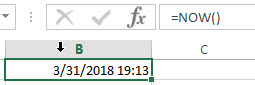2# get the date and time 12 hours ago, you can use the following formula:

`=NOW()-0.5`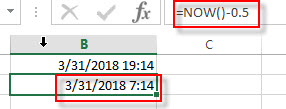3# get the date and time 5 days in the future, just use the following formula:

`=NOW() + 5`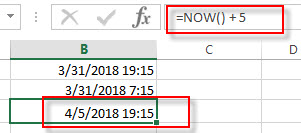### Related Functions

• Excel HOUR Function
The Excel HOUR function returns the hour of a time value. Or returns an integer value that represent the hour component of a given time. And the return value is between 0-23.The syntax of the HOUR function is as below:=HOUR (serial_number)…
• Excel SECOND Function
The Excel SECOND function returns the seconds of a time value. Or returns an integer value that represent the second component of a given excel time. And the return value is between 0-59.The syntax of the SECOND function is as below:=SECOND (serial_number)…
• Excel MINUTE Function
The Excel MINUTE function returns the minutes of a time value. And the minutes is an integer number from 0 to 59. The syntax of the MINUTE function is as below:=MINUTE (serial_number)…
• Excel DAY function
The Excel DAY function returns a day of a date (from 1 to 31). The DAY function is a build-in function in Microsoft Excel and it is categorized as a DATE and TIME Function.The syntax of the DAY function is as below:= DAY (date_value)
• Excel YEAR function
The Excel YEAR function returns a four-digit year from a given date value, the year is returned as an integer ranging from 1900 to 9999. The syntax of the YEAR function is as below:=YEAR (serial_number)…
• Excel MONTH function
The Excel MONTH function returns the month of a date represented by a serial number. And the month is an integer number from 1 to 12. The syntax of the MONTH function is as below:=MONTH (serial_number)…

## Excel MINUTE Function

This post will guide you how to use Excel MINUTE function with syntax and examples in Microsoft excel.

Description

The Excel MINUTE function returns the minutes of a time value. And the minutes is an integer number from 0 to 59. So you can use the MINUTE function to extract the minute component of a given time value.

For example, for a valid time value 10:29 AM, the MINUTE function will return 29. So you can pass the returned result into another formula.

The MINUTE function is a build-in function in Microsoft Excel and it is categorized as a DATE and TIME Function.

The MINUTE function is available in Excel 2016, Excel 2013, Excel 2010, Excel 2007, Excel 2003, Excel XP, Excel 2000, Excel 2011 for Mac.

### Syntax

The syntax of the MINUTE function is as below:

`=MINUTE (serial_number)`

Where the MINUTE function arguments is:

Serial_number -This is a required argument. A valid time value that contains the minute you want to extract. The times can be entered as a text strings within quotation marks (for example, “10:32 AM“), or typed as a decimal numbers, or the results of others formulas.

Note:

• Time values are a portion of a date value and represented by a decimal number (for example, 12:00 PM is represented as 0.5, since it is half of a day).
• If the serial_number is not a valid Excel time, it will return #VALUE! Error.

### Excel MINUTE Function Examples

The below examples will show you how to use Excel MINUTE Function to return an integer number that representing the minute component of a given Excel time.

1# get minute portion of the time, enter the following formula in Cell B1.

`=MINUTE(A1)`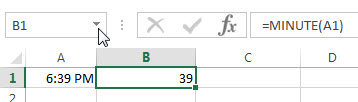### Related Functions

• Excel HOUR Function
The Excel HOUR function returns the hour of a time value. Or returns an integer value that represent the hour component of a given time. And the return value is between 0-23.The syntax of the HOUR function is as below:=HOUR (serial_number)…
• Excel SECOND Function
The Excel SECOND function returns the seconds of a time value. Or returns an integer value that represent the second component of a given excel time. And the return value is between 0-59.The syntax of the SECOND function is as below:=SECOND (serial_number)…
• Excel DAY function
The Excel DAY function returns a day of a date (from 1 to 31). The DAY function is a build-in function in Microsoft Excel and it is categorized as a DATE and TIME Function.The syntax of the DAY function is as below:= DAY (date_value)
• Excel YEAR function
The Excel YEAR function returns a four-digit year from a given date value, the year is returned as an integer ranging from 1900 to 9999. The syntax of the YEAR function is as below:=YEAR (serial_number)…
• Excel MONTH function
The Excel MONTH function returns the month of a date represented by a serial number. And the month is an integer number from 1 to 12. The syntax of the MONTH function is as below:=MONTH (serial_number)…
• Excel TIMEVALUE Function
The Excel TIMEVALUE function returns the decimal number of the time represented by a text string. so it will convert a time represented by a text string into an Excel time value.The syntax of the TIMEVALUE function is as below:=TIMEVALUE (time_text)…

## Excel SECOND Function

This post will guide you how to use Excel SECOND function with syntax and examples in Microsoft excel.

### Description

The Excel SECOND function returns the seconds of a time value. Or returns an integer value that represent the second component of a given excel time. And the return value is between 0-59.

For example, if you pass a time of 6:32:54 PM into SECOND function, and it will return 54. So you can use this function to extract the second component from a time value.

The SECOND function is a build-in function in Microsoft Excel and it is categorized as a DATE and TIME Function.

The SECOND function is available in Excel 2016, Excel 2013, Excel 2010, Excel 2007, Excel 2003, Excel XP, Excel 2000, Excel 2011 for Mac.

### Syntax

The syntax of the SECOND function is as below:

`=SECOND (serial_number)`

Where the SECOND function arguments is:

Serial_text -This is a required argument. The time from which you want to extract the second component. it may be typed as text string within quotation marks (for example, “13:52 PM”), or it can be a decimal numbers(for example, 0.5), or the result of a formula (for example, TIMEVALUE(“13.54:30 PM”))

Note:

• A serial date is how the Microsoft Excel stores dates and times and it represents the number of days since 1900-01-01, so the January 1, 1900 date is serial number 1 by default.
• If the serial_number is not a valid Excel time, it will return #VALUE! Error.

### Excel SECOND Function Examples

The below examples will show you how to use Excel SECOND Function to return the seconds of a given time value.

1# get the seconds from an Excel time 6:38:45, enter the following formula in Cell B1.

`=SECOND(6:38:45)`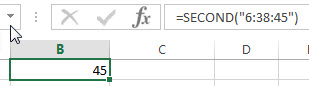2# Get the second portion of the date with no second portion specified, enter the following formula in Cell B3.

`=SECOND("6:38")`

or

`=SECOND(TIMEVALUE("6:38 PM"))`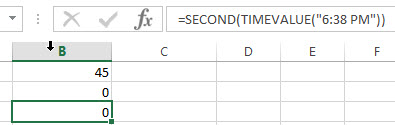It will return 0.

### Related Functions

• Excel HOUR Function
The Excel HOUR function returns the hour of a time value. Or returns an integer value that represent the hour component of a given time. And the return value is between 0-23.The syntax of the HOUR function is as below:=HOUR (serial_number)…
• Excel MINUTE Function
The Excel MINUTE function returns the minutes of a time value. And the minutes is an integer number from 0 to 59. The syntax of the MINUTE function is as below:=MINUTE (serial_number)…
• Excel DAY function
The Excel DAY function returns a day of a date (from 1 to 31). The DAY function is a build-in function in Microsoft Excel and it is categorized as a DATE and TIME Function.The syntax of the DAY function is as below:= DAY (date_value)
• Excel YEAR function
The Excel YEAR function returns a four-digit year from a given date value, the year is returned as an integer ranging from 1900 to 9999. The syntax of the YEAR function is as below:=YEAR (serial_number)…
• Excel MONTH function
The Excel MONTH function returns the month of a date represented by a serial number. And the month is an integer number from 1 to 12. The syntax of the MONTH function is as below:=MONTH (serial_number)…
• Excel TIMEVALUE Function
The Excel TIMEVALUE function returns the decimal number of the time represented by a text string. so it will convert a time represented by a text string into an Excel time value.The syntax of the TIMEVALUE function is as below:=TIMEVALUE (time_text)…

## Excel DATEVALUE Function

This post will guide you how to use Excel DATEVALUE function with syntax and examples in Microsoft excel.

### Description

The Excel DATEVALUE function returns the serial number of date. And it can be used to convert a date represented as text format into a serial number that recognizes as a date format.

For example, you can convert a date “2018/03/04” that is text format to a serial number, you can use the formula: =DATEVALUE (“2018/03/04”). Then it returns a serial number “43163” you can use the Excel dates to manipulate with formulas or pivot table to filter, sort. So it is very useful than the text dates.

The DATEVALUE function is a build-in function in Microsoft Excel and it is categorized as a DATE and TIME Function.

The DATEVALUE function is available in Excel 2016, Excel 2013, Excel 2010, Excel 2007, Excel 2003, Excel XP, Excel 2000, Excel 2011 for Mac.

### Syntax

The syntax of the DATEVALUE function is as below:

`=DATEVALUE(date_text)`

Where the EDATE function arguments is:

• Date_text -This is a required argument. A valid date that the format is text. Or the text that represents a date in an excel date format.

Note:

• The date_text argument must represent a date between January 1, 1900 and December 31, 9999. The DATEVALUE function returns the #VALUE! Error value if the value of the date_text argument falls outside of this range.
• If the year portion of the date_text argument is omitted, the DATEVALUE function uses the current year from your computer’s built-in clock. Time information in the date_text argument is ignored.
• January 1 1900 is serial number 1 by default.

### Excel DATEVALUE Function Examples

The below examples will show you how to use Excel DATEVALUE Function to return a serial number of a given date.

1# Get a serial number of a date (03/14/2018) the format as text, enter the following formula in Cell B1.

`=DATEVALUE("03/14/2018")`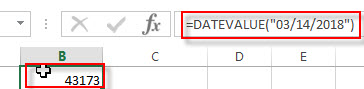2# Get a serial number of a date (14-May-2018) the format as text, enter the following formula in Cell B2.

`=DATEVALUE("14-May-2018")`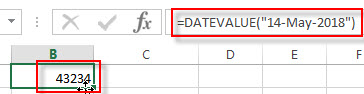3# Get a serial number of a date (2018/03/14) the format as text, enter the following formula in Cell B3.

`=DATEVALUE("2018/03/14")`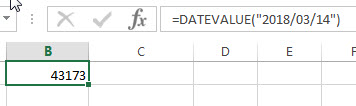4# get a serial number from a date that the year portion is omitted (03/04) assuming the current year is 2018, using the following formula:

`=DATEVALUE("03/14")`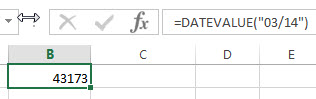5# get a serial number from a date that created by combining the values in Cells A1, A2, and A3

`=DATEVALUE(A1&"/"&A2&"/"&A3)`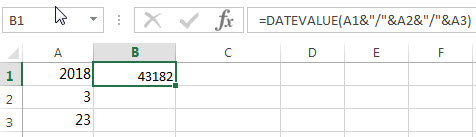### Related Functions

• Excel DAY function
The Excel DAY function returns a day of a date (from 1 to 31). The DAY function is a build-in function in Microsoft Excel and it is categorized as a DATE and TIME Function.The syntax of the DAY function is as below:= DAY (date_value)
• Excel YEAR function
The Excel YEAR function returns a four-digit year from a given date value, the year is returned as an integer ranging from 1900 to 9999. The syntax of the YEAR function is as below:=YEAR (serial_number)…
• Excel MONTH function
The Excel MONTH function returns the month of a date represented by a serial number. And the month is an integer number from 1 to 12. The syntax of the MONTH function is as below:=MONTH (serial_number)…

### More Excel Datevalue Function Examples

• Convert Month Name to Number
If you want to convert month name to number with an excel formula, or you want to convert 3 letter month name to numbers, you can create a formual based on the MONTH function and the DATEVALUE function…
• Compare Dates
Assuming that you have a list of data that contain date values in Excel, you can use the IF function to create a formula to achieve it. If the date is greater that the given date value, then return True. Otherwise, it returns False….

## Excel MONTH Function

This post will guide you how to use Excel MONTH function with syntax and examples in Microsoft excel.

### Description

The Excel MONTH function returns the month of a date represented by a serial number. And the month is an integer number from 1 to 12. And you can use the MONTH function to get a month component from a given date value. And you can feed the month number to another formula.

The MONTH function is a build-in function in Microsoft Excel and it is categorized as a DATE and TIME Function.

The MONTH function is available in Excel 2016, Excel 2013, Excel 2010, Excel 2007, Excel 2003, Excel XP, Excel 2000, Excel 2011 for Mac.

### Syntax

The syntax of the MONTH function is as below:

`=MONTH (serial_number)`

Where the MONTH function arguments is:

• Serial_number -This is a required argument. A valid date value that contains the month component you want to extract. Dates should be entered by using the DATE function, or as results of other formulas or functions. For example, use DATE (2019,3,15) for the 15th day of March, 2019.

Note:

• A serial date is how the Microsoft Excel stores dates and it represents the number of days since 1900-01-01, so the January 1, 1900 date is serial number 1 by default.
• If the serial_number is not a valid Excel date, it will return #VALUE! Error.

### Excel MONTH Function Examples

The below examples will show you how to use Excel MONTH Function to return an integer number that representing the month component of a given Excel date

1# get month of the date in Cell A1, enter the following formula in Cell B1.

`=MONTH(A1)`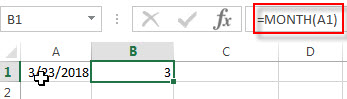2# convert Month name to number, type the following formula in Cell B1.

You should use the DATEVALUE function to convert a date stored as text to a serial number. Then feed the result into the MONTH function to extract a month number from date.

`=MONTH(DATEVALUE(A1 & "1"))`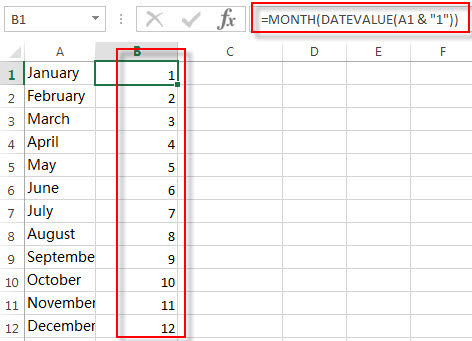### Related Functions

• Excel DAY function
The Excel DAY function returns a day of a date (from 1 to 31). The DAY function is a build-in function in Microsoft Excel and it is categorized as a DATE and TIME Function.The syntax of the DAY function is as below:= DAY (date_value)
• Excel YEAR function
The Excel YEAR function returns a four-digit year from a given date value, the year is returned as an integer ranging from 1900 to 9999. The syntax of the YEAR function is as below:=YEAR (serial_number)…
• Excel DATEVALUE Function
The Excel DATEVALUE function returns the serial number of date. And it can be used to convert a date represented as text format into a serial number that recognizes as a date format.The syntax of the DATEVALUE function is as below:=DATEVALUE(date_text)…

### More Excel Month Function Examples

• Convert Month Name to Number
If you want to convert month name to number with an excel formula, or you want to convert 3 letter month name to numbers, you can create a formual based on the MONTH function and the DATEVALUE function…
• Count Dates in Given Year/Month/Day in Excel
You can create a formula based on the SUMPRODUCT function and the YEAR function to count dates by a give year….

## Excel TODAY Function

This post will guide you how to use Excel TODAY function with syntax and examples in Microsoft excel.

### Description

The Excel TODAY function returns the serial number of the current date. So you can get the current system date from the TODAY function. The serial number is the date-time code used by Excel for date and time calculations.

You can use the TODAY function to get the current date displayed on your worksheet, and regardless of when you open the workbook. And it also can be used for calculating intervals. For example, if you want to know that person’s age (he was born in 1984), you can use the following formula:

=YEAR(TODAY())-1984

This formula will use the TODAY function as an argument for the YEAR function to get the current year, and then subtracts 1984, getting his age.

The TODAY function is a build-in function in Microsoft Excel and it is categorized as a DATE and TIME Function.

The TODAY function is available in Excel 2016, Excel 2013, Excel 2010, Excel 2007, Excel 2003, Excel XP, Excel 2000, Excel 2011 for Mac.

### Syntax

The syntax of the TODAY function is as below:

`=TODAY()`

There are no any arguments for the TODAY function, but you still need to use empty parentheses ().

### Excel TODAY Function Examples

The below examples will show you how to use Excel TODAY Function to return the current system date.

1# using the TODAY function to get the current date, enter the following formula in Cell B1.

`=TODAY()`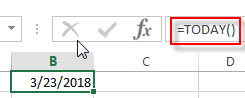2# Get the current date and then subtracts 10 days, using the following formula:

`=TODAY() -10`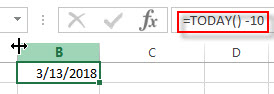3# Get the number of days between the current date and 03/14/2019, type the following formula:

`=DATEVALUE("03/14/2019")-TODAY()`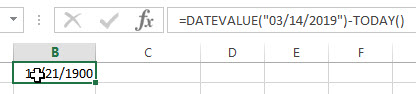4# Get the current day of the month, using the following formula:

`=DAY(TODAY())`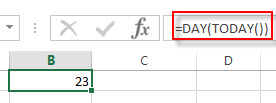5# Get the current month of the year, type the following formula in Cell B1:

`=MONTH(TODAY())`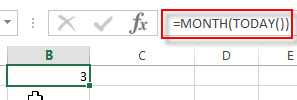You can press F9 key to force the worksheet to recalculate each time and then update the value. If the TODAY function does not update the date when you expect it to, you might need to change the settings that control when the workbook or worksheet recalculates. On the File tab, click Options, and then in the Formulas category under Calculation options, make sure that Automatic is selected.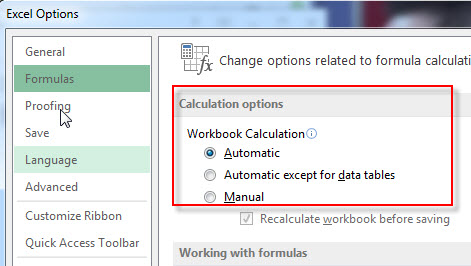### Related Functions

• Excel DAY function
The Excel DAY function returns a day of a date (from 1 to 31). The DAY function is a build-in function in Microsoft Excel and it is categorized as a DATE and TIME Function.The syntax of the DAY function is as below:= DAY (date_value)
• Excel YEAR function
The Excel YEAR function returns a four-digit year from a given date value, the year is returned as an integer ranging from 1900 to 9999. The syntax of the YEAR function is as below:=YEAR (serial_number)…
• Excel MONTH function
The Excel MONTH function returns the month of a date represented by a serial number. And the month is an integer number from 1 to 12. The syntax of the MONTH function is as below:=MONTH (serial_number)…
• Excel DATEVALUE Function
The Excel DATEVALUE function returns the serial number of date. And it can be used to convert a date represented as text format into a serial number that recognizes as a date format.The syntax of the DATEVALUE function is as below:=DATEVALUE(date_text)…

### More Excel TODAY Function Examples

• Conditional Formatting date with red Amber or Green
How to highlight the date with red if the cell dates is past now(). How to highlight the date with amber if the cell date is past now but within the next 6 months from now(). How to highlight the date with green if the cell date is more than 6 months from now…
• Highlight Rows If Dates Have Passed
Assuming that you have a worksheet that have a bunch of columns and each row has a date column. If the date in the date column goes past from now on and you want to highlight the entire row with red color….
• Count Dates in Given Year/Month/Day in Excel
You can create a formula based on the SUMPRODUCT function and the YEAR function to count dates by a give year….

## Excel YEAR Function

This post will guide you how to use Excel YEAR function with syntax and examples in Microsoft excel.

### Description

The Excel YEAR function returns a four-digit year from a given date value, the year is returned as an integer ranging from 1900 to 9999. So you can use the YEAR function to get the year component of a date. You can also use this function to extract the year portion from an Excel date and feed the returned value into another formula.

The YEAR function is a build-in function in Microsoft Excel and it is categorized as a DATE and TIME Function.

The YEAR function is available in Excel 2016, Excel 2013, Excel 2010, Excel 2007, Excel 2003, Excel XP, Excel 2000, Excel 2011 for Mac.

### Syntax

The syntax of the YEAR function is as below:

`=YEAR (serial_number)`

Where the YEAR function arguments is:

• Serial_number -This is a required argument. A valid date value that contains the year component you want to extract. Dates should be entered by using the DATE function, or as results of other formulas or functions. For example, use DATE(2019,3,15) for the 15th day of March, 2019.

Note:

• A serial date is how the Microsoft Excel stores dates and it represents the number of days since 1900-01-01, so the January 1, 1900 date is serial number 1 by default.
• If the serial_number is not a valid Excel date, it will return #VALUE! Error.

### Examples

The below examples will show you how to use Excel YEAR Function to return an integer number that representing the year component of a given Excel date

1# get year of the date in Cell A1 , enter the following formula in Cell B1.

=YEAR(A1)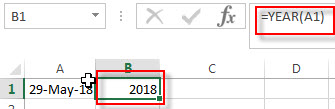### Related Functions

• Excel WEEKDAY function
The Excel WEEKDAY function returns a integer value representing the day fo the week for a given Excel date and the value is range from 1 to 7.The syntax of the WEEKDAY function is as below:=WEEKDAY (serial_number,[return_type])…
• Excel DAY function
The Excel DAY function returns a day of a date (from 1 to 31).The DAY function is a build-in function in Microsoft Excel and it is categorized as a DATE and TIME Function.The syntax of the DAY function is as below:= DAY (date_value)…
• Excel DATEVALUE Function
The Excel DATEVALUE function returns the serial number of date. And it can be used to convert a date represented as text format into a serial number that recognizes as a date format.The syntax of the DATEVALUE function is as below:=DATEVALUE(date_text)…
• Excel MONTH function
The Excel MONTH function returns the month of a date represented by a serial number. And the month is an integer number from 1 to 12. The syntax of the MONTH function is as below:=MONTH (serial_number)…

## Excel WORKDAY.INTL Function

This post will guide you how to use Excel WORKDAY.INTL function with syntax and examples in Microsoft excel.

### Description

The Excel WORKDAY.INTL function returns the serial number of the date before or after a specified number of workdays. And this function allow you to exclude weekends and holidays as non-working days. You can use the WORKDAY.INTL function to calculate working days excluding weekdays and holidays.

The WORKDAY.INTL function is a build-in function in Microsoft Excel and it is categorized as a DATE and TIME Function.

The WORKDAY.INTL function is available in Excel 2016, Excel 2013, Excel 2010, Excel 2007, Excel 2003, Excel XP, Excel 2000, Excel 2011 for Mac.

### Syntax

The syntax of the WORKDAY.INTL function is as below:

`= WORKDAY.INTL (start_date, days, [weekend], [holidays])`

Where the WORKDAY.INTL function arguments is:

• Start_date –This is a required argument. The starting date from which you want to count the number of working days. The date should be typed as a valid Excel time a serial date.
• days – This is a required argument. The number of working days that you want to add. A positive value for days yields a future date; a negative value yields a past date.
• Weekend – This is an optional argument. Determine which days of the week that are weekend days and are not considered working days. Weekend is a weekend number and it can be one of the following numbers.
 weekend-number Weekend days 1 or omitted Saturday, Sunday 2 Sunday, Monday 3 Monday, Tuesday 4 Tuesday, Wednesday 5 Wednesday, Thursday 6 Thursday, Friday 7 Friday, Saturday 11 Sunday only 12 Monday only 13 Tuesday only 14 Wednesday only 15 Thursday only 16 Friday only 17 Saturday only
• holidays – This is an optional argument. The list of holidays that you want to exclude from the working days. It can be a range of cells that contain the holiday dates or it can be a list of serial numbers that represent the holiday dates.

Note:

• If any argument is not a valid Excel date, a #VALUE! Error is returned.
• If start date value plus days is an invalid date, the WORKDAY function returns #NUM! Error.
• A serial date is how the Microsoft Excel stores dates and it represents the number of days since 1900-01-01, so the January 1, 1900 date is serial number 1 by default.
• If days value is not a integer number, it will be truncated.
• If a weekend string is of invalid length or contains invalid characters, WORKDAY.INTL returns the #VALUE! Error value.

### Excel WORKDAY.INTL Function Examples

The below examples will show you how to use Excel WORKDAY.INTL Function to return the working days from the start date.

1# get the date 50 workdays from the starting date “3/20/2018”, counting Sunday and Monday as a weekend day, so the weekend argument should be 2, enter the following formula in Cell B1.

`=WORKDAY.INTL(DATE(2018,3,20),50,2)`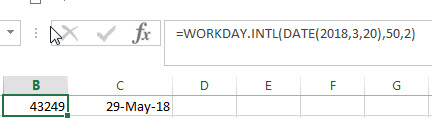2# get the date 50 workdays from the starting date “3/20/2018”, excluding holidays 5/1/2018, and counting only Sundays as weekend day, the weekend argument should be 11, type the following formula in Cell B2.

`=WORKDAY(DATE(2018,3,20),50,11,DATE(2018,5,1))`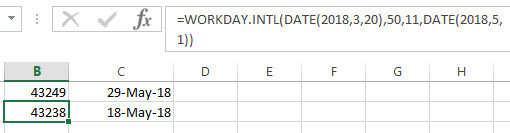### Related Functions

• Excel WORKDAY function
The Excel WORKDAY function returns a serial number that represents a date that is the indicated number of working days before or after the starting date you specified.The syntax of the WORKDAY function is as below:=WORKDAY(start_date, days, [holidays])…
• Excel WEEKDAY function
The Excel WEEKDAY function returns a integer value representing the day fo the week for a given Excel date and the value is range from 1 to 7.The syntax of the WEEKDAY function is as below:=WEEKDAY (serial_number,[return_type])…
• Excel DAY function
The Excel DAY function returns a day of a date (from 1 to 31).The DAY function is a build-in function in Microsoft Excel and it is categorized as a DATE and TIME Function.The syntax of the DAY function is as below:= DAY (date_value)…
• Excel DATE function
The Excel DATE function returns the serial number for a date.The syntax of the DATE function is as below:= DATE (year, month, day)…

## Excel WORKDAY Function

This post will guide you how to use Excel WORKDAY function with syntax and examples in Microsoft excel.

### Description

The Excel WORKDAY function returns a serial number that represents a date that is the indicated number of working days before or after the starting date you specified. So you can add a specified number of working days to the starting date and then returns a serial date. The working days will exclude weekends and any dates specified as holidays.

You can use the WORKDAY function to calculate working days and non-working days.

The WORKDAY function is a build-in function in Microsoft Excel and it is categorized as a DATE and TIME Function.

The WORKDAY function is available in Excel 2016, Excel 2013, Excel 2010, Excel 2007, Excel 2003, Excel XP, Excel 2000, Excel 2011 for Mac.

### Syntax

The syntax of the WORKDAY function is as below:

`=WORKDAY(start_date, days, [holidays])`

Where the WORKDAY function arguments is:

• Start_date –This is a required argument. The starting date from which you want to count the number of working days. The date should be typed as a valid Excel time a serial date.
• days – This is a required argument. The number of working days that you want to add. A positive value for days yields a future date; a negative value yields a past date.
• holidays – This is an optional argument. The list of holidays that you want to exclude from the working days. It can be a range of cells that contain the holiday dates or it can be a list of serial numbers that represent the holiday dates.

Note:

• If any argument is not a valid Excel date, a #VALUE! Error is returned.
• If start date value plus days is an invalid date, the WORKDAY function returns #NUM! Error.
• A serial date is how the Microsoft Excel stores dates and it represents the number of days since 1900-01-01, so the January 1, 1900 date is serial number 1 by default.
• If days value is not a integer number, it will be truncated.

### Excel WORKDAY Function Examples

The below examples will show you how to use Excel WORKDAY Function to return the working days from the start date.

1# get the date 50 workdays from the starting date “3/20/2018”, enter the following formula in Cell B1.

`=WORKDAY(DATE(2018,3,20),50)`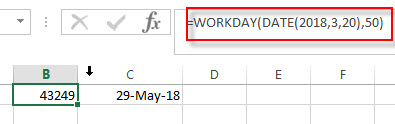2# get the date 50 workdays from the starting date “3/20/2018”, excluding holidays 5/1/2018, type the following formula in Cell B2.

`=WORKDAY(DATE(2018,3,20),50,DATE(2018,5,1))`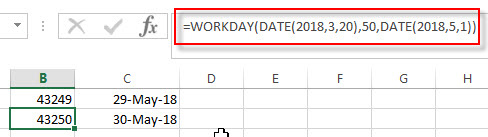### Related Functions

• Excel WEEKDAY function
The Excel WEEKDAY function returns a integer value representing the day fo the week for a given Excel date and the value is range from 1 to 7.The syntax of the WEEKDAY function is as below:=WEEKDAY (serial_number,[return_type])…
• Excel DAY function
The Excel DAY function returns a day of a date (from 1 to 31).The DAY function is a build-in function in Microsoft Excel and it is categorized as a DATE and TIME Function.The syntax of the DAY function is as below:= DAY (date_value)…
• Excel DATE function
The Excel DATE function returns the serial number for a date.The syntax of the DATE function is as below:= DATE (year, month, day)…

### More Excel Workday Function Examples

• Calculating Future Date
If you want to calculate a new future date excluding weekends, and you need to use the WORKDAY function to create a new formula to exclude all weekends in the future date.…

## Excel WEEKNUM function

This post will guide you how to use Excel WEEKNUM function with syntax and examples in Microsoft excel.

### Description

The Excel WEEKNUM function returns the week number of a specific date, and the returned value is ranging from 1 to 53. And this function will start to count the week number from January 1 and the Sunday is the first day in one week by default.

The WEEKNUM function is a build-in function in Microsoft Excel and it is categorized as a DATE and TIME Function.

The WEEKNUM function is available in Excel 2016, Excel 2013, Excel 2010, Excel 2007, Excel 2003, Excel XP, Excel 2000, Excel 2011 for Mac.

### Syntax

The syntax of the WEEKNUM function is as below:

`=WEEKNUM (serial_number,[return_type])`

Where the WEEKNUM function arguments is:

• Serial_number –This is a required argument. An Excel date that you want to get the week number. The dates should be typed by the DATE function to make sure it is a valid Excel date. For example, use DATE(2018,3,20) to pass into the WEEKNUM function and then it will return the week number.
• return_type – This is an optional argument. A number that determines the first day of the week for the year, the default value is 1.
 Return_type Week begins on System 1 or omitted Sunday 1 2 Monday 1 11 Monday 1 12 Tuesday 1 13 Wednesday 1 14 Thursday 1 15 Friday 1 16 Saturday 1 17 Sunday 1 21 Monday 2

Note:

• If serial_number is out of range for the current date base value, a #NUM! Error is returned.
• If return_type is out of the range specified in the table above, a #NUM! Error is returned.
• A serial date is how the Microsoft Excel stores dates and it represents the number of days since 1900-01-01, so the January 1, 1900 date is serial number 1 by default.

### Excel WEEKNUM Function Examples

The below examples will show you how to use Excel WEEKNUM Function to return the week number from an Excel date.

1# get number of the week from a date value 2018/03/20, enter the following formula in Cell B1.

=WEEKNUM(DATE(2018,3,2018))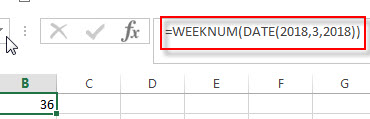### Related Functions

• Excel WEEKDAY function
The Excel WEEKDAY function returns a integer value representing the day fo the week for a given Excel date and the value is range from 1 to 7.The syntax of the WEEKDAY function is as below:=WEEKDAY (serial_number,[return_type])…
• Excel WORKDAY function
The Excel WORKDAY function returns a serial number that represents a date that is the indicated number of working days before or after the starting date you specified.The syntax of the WORKDAY function is as below:=WORKDAY(start_date, days, [holidays])…
• Excel DAY function
The Excel DAY function returns a day of a date (from 1 to 31).The DAY function is a build-in function in Microsoft Excel and it is categorized as a DATE and TIME Function.The syntax of the DAY function is as below:= DAY (date_value)…
• Excel EDATE function
TThe Excel EDATE function returns the serial number that represents the date that is a specified number of months before or after a specified date.The syntax of the EDATE function is as below:=EDATE (start_date, months)…
• Excel DATE function
The Excel DATE function returns the serial number for a date.The syntax of the DATE function is as below:= DATE (year, month, day)…

### More Excel WEEKNUM Function Examples

• Converting Week Number to Date
You need to create a formula based on the MAX function, the DATE function and the WEEKDAY function to convert week number to a date in Excel.…

## Excel WEEKDAY Function

This post will guide you how to use Excel WEEKDAY function with syntax and examples in Microsoft excel.

### Description

The Excel WEEKDAY function returns a integer value representing the day fo the week for a given Excel date and the value is range from 1 to 7. so you can use this function to get the weekday number for a supplied date. and it will returns 1 for Sunday and returns 7 for Saturday. you also can use the WEEKDAY function to combine with other formulas or functions.

The WEEKDAY function is a build-in function in Microsoft Excel and it is categorized as a DATE and TIME Function.

The WEEKDAY function is available in Excel 2016, Excel 2013, Excel 2010, Excel 2007, Excel 2003, Excel XP, Excel 2000, Excel 2011 for Mac.

### Syntax

The syntax of the WEEKDAY function is as below:

`=WEEKDAY (serial_number,[return_type])`

Where the WEEKDAY function arguments is:

• Serial_number –This is a required argument. An Excel date that you want to get the weekday of. the dates should be typed by the DATE function to make sure it is a valid Excel date. For example, use DATE(2018,3,20) to pass into the WEEKDAY function and then it will return the weekday of this date.
• return_type – This is an optional argument. A number that determines the type of return value.
 Return_type Number returned 1 or omitted Numbers 1 (Sunday) through 7 (Saturday). Behaves like previous versions of Microsoft Excel. 2 Numbers 1 (Monday) through 7 (Sunday). 3 Numbers 0 (Monday) through 6 (Sunday). 11 Numbers 1 (Monday) through 7 (Sunday). 12 Numbers 1 (Tuesday) through 7 (Monday). 13 Numbers 1 (Wednesday) through 7 (Tuesday). 14 Numbers 1 (Thursday) through 7 (Wednesday). 15 Numbers 1 (Friday) through 7 (Thursday). 16 Numbers 1 (Saturday) through 7 (Friday). 17 Numbers 1 (Sunday) through 7 (Saturday).

Note:

• If serial_number is out of range for the current date base value, a #NUM! error is returned.
• If return_type is out of the range specified in the table above, a #NUM! error is returned.
• A serial date is how the Microsoft Excel stores dates and it represents the number of days since 1900-01-01, so the January 1, 1900 date is serial number 1 by default.

### Excel WEEKDAY Function Examples

The below examples will show you how to use Excel WEEKDAY Function to return a weekday of a date.

1# get day of the week with the default return type 1, enter the following formula in Cell B1.

=WEEKDAY(DATE(2018,3,20))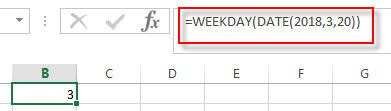it means that the weekday of that date is Tuesday.

### Related Functions

• Excel WEEKNUM function
The Excel WEEKNUM function returns the week number of a specific date, and the returned value is ranging from 1 to 53.The syntax of the WEEKNUM function is as below:=WEEKNUM (serial_number,[return_type])…
• Excel DAY function
The Excel DAY function returns a day of a date (from 1 to 31).The DAY function is a build-in function in Microsoft Excel and it is categorized as a DATE and TIME Function.The syntax of the DAY function is as below:= DAY (date_value)…
• Excel EDATE function
TThe Excel EDATE function returns the serial number that represents the date that is a specified number of months before or after a specified date.The syntax of the EDATE function is as below:=EDATE (start_date, months)…
• Excel DATE function
The Excel DATE function returns the serial number for a date.The syntax of the DATE function is as below:= DATE (year, month, day)…

### More Excel WEEKDAY Function Examples

• Calculate Thanksgiving Date
You can use a simple arithmetic to calculate the possible date range for the US Thanksgiving, it falls on the fourth Thursday in November. And you can create a formula based on the DATE function and the WEEKDAY function.…
• Convert Date to Day of Week in Excel (get day name from date )
If you want to get the day name from a date in excel, you can use the TEXT function with a specified format code like “ddd” or “dddd”..…
• Get the First Monday of a Given Year
To calculate the first Monday of the year or given any date, you can create a new complex formula based on the DATE function, the YEAR function and the WEEKDAY function……
• Converting Week Number to Date
You need to create a formula based on the MAX function, the DATE function and the WEEKDAY function to convert week number to a date in Excel.…

## Excel TIMEVALUE Function

This post will guide you how to use Excel TIMEVALUE function with syntax and examples in Microsoft excel.

### Description

The Excel TIMEVALUE function returns the decimal number of the time represented by a text string. so it will convert a time represented by a text string into an Excel time value. and the returned value is range from 0 to 0.99988, it indicated the times from 0:00:00 to 23:59:59.
For example, when you fed the text string “7:13 PM” into TIMEVALUE function, and it returns 0.80069. the returned value is a numeric value and this value can be used to manipulate with another formulas or pivot tables.

The TIMEVALUE function is a build-in function in Microsoft Excel and it is categorized as a DATE and TIME Function.

The TIMEVALUE function is available in Excel 2016, Excel 2013, Excel 2010, Excel 2007, Excel 2003, Excel XP, Excel 2000, Excel 2011 for Mac.

### Syntax

The syntax of the TIMEVALUE function is as below:

`=TIMEVALUE (time_text)`

Where the TIMEVALUE function arguments is:

• Serial_text -This is a required argument. A text string that represents a time, within the text string, the hours, minutes and seconds components should be separated by colon characters.

Note:

• the date information in text string will be ignored.
• if the time_textr is not a valid Excel time in a text format , it will return #VALUE! error.

### Excel TIMEVALUE Function Examples

The below examples will show you how to use Excel TIMEVALUE Function to convert a text string that representing a time in Excel.

1# get decimal part of a day, with only the time portion specified, enter the following formula in Cell B1.

`=TIMEVALUE("7:27 PM")`

2# get decimal part of a day, with date and time specified, enter the following formula in Cell B3.

``=TIMEVALUE("19-March-2018 7:28PM") ``

## Excel TIME Function

This post will guide you how to use Excel TIME function with syntax and examples in Microsoft excel.

### Description

The Excel TIME function returns a date in serial number format for a particular time (the hour, minute and second).

The serial number returned by TIME function is a value between 0 and 0.99988426. And the result of 0 represents 12:00:00 AM and the result of 0.99988426 represents 11:59:59 PM.

So this function can be used to create a time with hours, minutes, and seconds. And it also will return a decimal number representing a particular time in excel.

The TIME function is a build-in function in Microsoft Excel and it is categorized as a DATE and TIME Function.
The TIME function is available in Excel 2016, Excel 2013, Excel 2010, Excel 2007, Excel 2003, Excel XP, Excel 2000, Excel 2011 for Mac.

### Syntax

The syntax of the TIME function is as below:

`= TIME(hour, minute, second)`

Where the TIME function arguments are:

• Hour -This is a required argument. A number from 0 (zero) to 32767 representing the hour. If the value is greater than 23 and then it will be divided by 24 and the remainder will be treated as the hour value. For example, the TIME(28,0,0) is equal to TIME(4,0,0).
• Minute – This is a required argument. A number from 0 to 32767 representing the minute. If the minute value is greater than 59 and it will be converted to hours and minutes (it means that every 60 minutes will add one hour to hour value). For example, TIME(1,75,0) = TIME(2,15,0).
• Second – This is a required argument. A number from 0 to 32767 representing the second. If the second value is greater than 59 and it will be converted to hours, minutes, and seconds (it means that every 60 seconds will add 1 minute to minute value). For example, TIME(1,20,75) = TIME(1,21,15) .

Excel TIME Function Examples
The below examples will show you how to use Excel TIME function to create a decimal number representing a particular time.

#1 =TIME(A2,B2,C2)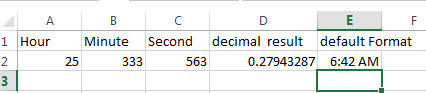## Excel EDATE Function

This post will guide you how to use Excel EDATE function with syntax and examples in Microsoft excel.

## Description

The Excel EDATE function returns the serial number that represents the date that is a specified number of months before or after a specified date. You can use the EDATE function to calculate the expiration dates or maturity dates or due dates that fall on the same day of the month as the date of issue. or you can add a specified number of months to a date.

The EDATE function is a build-in function in Microsoft Excel and it is categorized as a  DATE and TIME Function.

The EDATE function is available in Excel 2016, Excel 2013, Excel 2010, Excel 2007, Excel 2003, Excel XP, Excel 2000, Excel 2011 for Mac.

## Syntax

The syntax of the EDATE function is as below:

`=EDATE (start_date, months)`

Where the EDATE function arguments are:

• Start_date -This is a required argument. Adate that represents the start date.
• months – This is a required argument. the number of months before or after start_date. if you provide a positive number for months, it represents the future date; if it is a negative number, then it represents a past date.

Notes:

• Microsoft Excel stores dates as a serial number, it indicated the number of days since January 1,1900. and the date January 1,1900 is serial number 1.
• if start_date is not a valid date, the EDATE function will return the #VALUE! error.
• if months is not an integer, it is truncated.

## Examples

The below examples will show you how to use Excel EDATE Function to return the serial number.
1# set a positive number for months argument in the EDATE function

`=EDATE(B1,2)`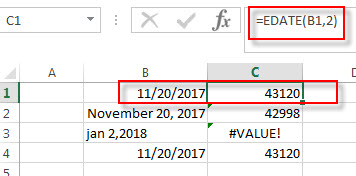2# set a negative number for months argument in the EDATE function, using the following formula:

`=EDATE(B2,-2)`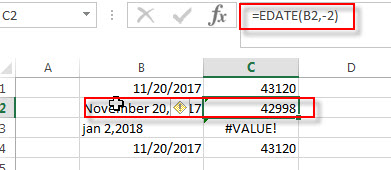3# set a invalid date for start_date argument in the EDATE function, type the following formula:

`=EDATE(B3,2)`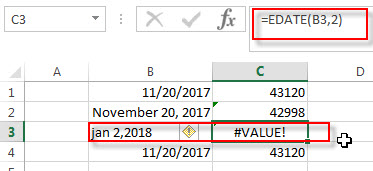4# set a value that is not an integer for months arguments, using the following formula:

`=EDATE(B4,2)`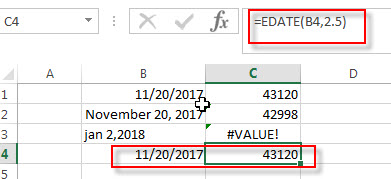### More Excel EDATE Function Examples

• Conditional Formatting date with red Amber or Green
How to highlight the date with red if the cell dates is past now(). How to highlight the date with amber if the cell date is past now but within the next 6 months from now(). How to highlight the date with green if the cell date is more than 6 months from now…

## Excel Days360 Function

This post will guide you how to use Excel DAYS360 function with syntax and examples in Microsoft excel.

### Description

The Excel DAYS360 function will calculate the number of days between two dates based on a 360-day year.

The DAYS360 function is a build-in function in Microsoft Excel and it is categorized as a  DATE and TIME Function.

The DAYS360 function is available in Excel 2016, Excel 2013, Excel 2010, Excel 2007, Excel 2003, Excel XP, Excel 2000, Excel 2011 for Mac.

### Syntax

The syntax of the DAYS360 function is as below:

=DAYS360 (start_date, end_date,[method])

Where the DAYS360 function arguments are:
Start_date and end_date -This is a required argument. The two dates to calculate the number of days.
Method – This is an optional argument.  It’s a Boolean value. If TRUE is set, the DAYS360 function will use the European method. Or it will use US method.

### Example

The below examples will show you how to use Excel DAYS360 Function to return the number of days between two dates based on a 360-day year.

#1 =DAYS360(B1,B2)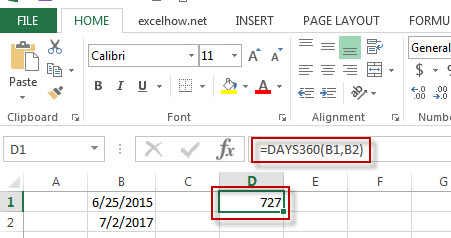Note: the above excel formula returns the number of days between 6/25/2015 and 7/2/2017, based on a 360-day year.

## Excel Days Function

This post will guide you how to use Excel DAYS function with syntax and examples in Microsoft excel.

### Description

The Excel DAYS function returns the number of days between two dates.

The DAYS function is a build-in function in Microsoft Excel and it is categorized as a DATE and TIME Function.

The DAYS function is available in Excel 2016, Excel 2013.

### Syntax

The syntax of the DAYS function is as below:

= DAYS (end_date,start_date)

### Example

The below examples will show you how to use Excel DAYS Function to return the number of days between end_date and start_date.

#1 =DAYS (B2,B1)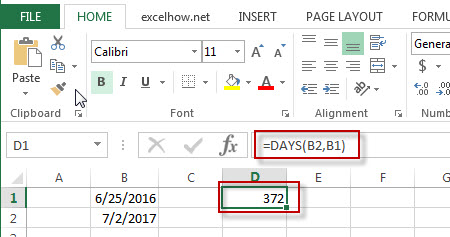Note: the above excel formula retruns the number of days (372) between the end date (7/2/2017) and start date (6/25/2016).

## Excel Day Function

This post will guide you how to use Excel DAY function with syntax and examples in Microsoft excel.

### Description

The Excel DAY function returns a day of a date (from 1 to 31)

The DAY function is a build-in function in Microsoft Excel and it is categorized as a DATE and TIME Function.

The DAY function is available in Excel 2016, Excel 2013, Excel 2010, Excel 2007, Excel 2003, Excel XP, Excel 2000, Excel 2011 for Mac.

### Syntax

The syntax of the DAY function is as below:

`= DAY (date_value)`

### Example

The below examples will show you how to use Excel DAY Function to return the day of a date.

#1 =DAY(B2)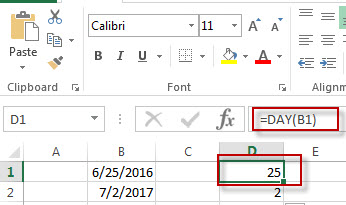Note:  The above excel formula will return the day of the date in Cell B1.

### Related Functions

• Excel YEAR function
The Excel YEAR function returns a four-digit year from a given date value, the year is returned as an integer ranging from 1900 to 9999. The syntax of the YEAR function is as below:=YEAR (serial_number)…
• Excel MONTH function
The Excel MONTH function returns the month of a date represented by a serial number. And the month is an integer number from 1 to 12. The syntax of the MONTH function is as below:=MONTH (serial_number)…
• Excel DATEVALUE Function
The Excel DATEVALUE function returns the serial number of date. And it can be used to convert a date represented as text format into a serial number that recognizes as a date format.The syntax of the DATEVALUE function is as below:=DATEVALUE(date_text)…
• Excel WEEKDAY function
The Excel WEEKDAY function returns a integer value representing the day fo the week for a given Excel date and the value is range from 1 to 7.The syntax of the WEEKDAY function is as below:=WEEKDAY (serial_number,[return_type])…

## Excel DatedIF Function

This post will guide you how to use Excel DATEDIF function with syntax and examples in Microsoft excel.

### Description

The Excel DATEDIF function returns the number of days, months, or years between tow dates.

The DATEDIF function is a build-in function in Microsoft Excel and it is categorized as a DATE and TIME Function.

The DATEDIF function is available in Excel 2016, Excel 2013, Excel 2010, Excel 2007, Excel 2003, Excel XP, Excel 2000, Excel 2011 for Mac.

### Syntax

The syntax of the DATEDIF function is as below:

=DATEDIF (start_date,end_date,unit)

Where the DATEDIF function arguments are:
Unit –  the type of information that you want to return.
Note: The index_num value must be a number between 1 and 29.

• “Y”– The number of complete years
• “M”– The number of complete months
• “D”– The number of days
• “MD”– The difference between the days in start_date and end_date.
• “YM”– The difference between the months in start_date and end_date
• “YD”– The difference between the days(the years of dates are ignored)

### Example

The below examples will show you how to use Excel DATEDIF Function to return the difference between two dates.

#1 =DATEDIF(B1,B2,”Y”)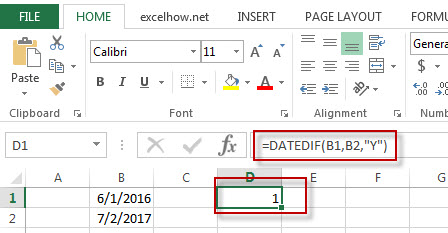Note: the above excel formula will return the number of complete year as “1”between start_date(6/1/2016) and end_date(07/2/2017).

#2 =DATEDIF(B1,B2,”D”)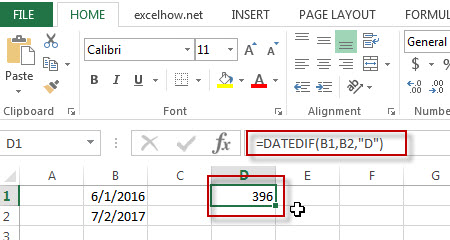Note: the above formula will return the number of days(396) between 6/1/2016 and 7/2/2017.

#3 =DATEDIF(B1,B2,”YD”)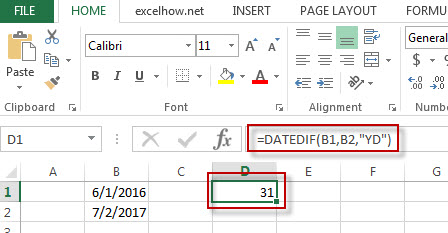Note: the above formula will return 31 days between 6/1 and 7/2, ignoring the years of dates.

## Excel Date Function

This post will guide you how to use Excel DATE function with syntax and examples in Microsoft excel.

### Description

The Excel DATE function returns the serial number for a date.

The DATE function is a build-in function in Microsoft Excel and it is categorized as a DATE and TIME Function.

The DATE function is available in Excel 2016, Excel 2013, Excel 2010, Excel 2007, Excel 2003, Excel XP, Excel 2000, Excel 2011 for Mac.

### Syntax

The syntax of the DATE function is as below:

```= DATE (year, month, day)
```

Note:

• If the year value is between 0 and 1899, excel will add that value to 1900 to determine the year.
• If the year value is between 1900 and 9999, excel will use that value as the year value.
• If year is less than 0 or is greater than 9999, excel will return the error value: #NUM!
• If month is greater than 12, every 12 months will add 1 year to the year value.
• If the day value is greater than the number of days in the month specified, then it will add that number of days to the first day in the month. For example: DATA(2017,6,32), the DATE function will return “7/2/2017”

### Example

The below examples will show you how to use Excel DATE Function to return the sequential serial number for a date.

#1 =DATE(A2,B2,C2)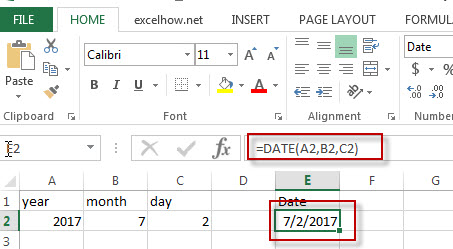### More Excel DATE Function Examples

• Calculate Thanksgiving Date
You can use a simple arithmetic to calculate the possible date range for the US Thanksgiving, it falls on the fourth Thursday in November. And you can create a formula based on the DATE function and the WEEKDAY function.…
• Get the First Monday of a Given Year
To calculate the first Monday of the year or given any date, you can create a new complex formula based on the DATE function, the YEAR function and the WEEKDAY function……
• Convert Month Name to Number
If you want to convert month name to number with an excel formula, or you want to convert 3 letter month name to numbers, you can create a formual based on the MONTH function and the DATEVALUE function…
• Converting Week Number to Date
You need to create a formula based on the MAX function, the DATE function and the WEEKDAY function to convert week number to a date in Excel.…